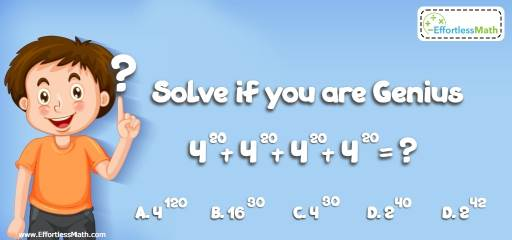# Number Properties Puzzle – Challenge 18

This is a great math challenge that hones students' logic and creative thinking skills the fun way.## Challenge:

$$4^{20}+4^{20}+4^{20}+4^{20} =$$ ?

A- $$4^{120}$$

B- $$16^{30}$$

C- $$4^{30}$$

D- $$2^{40}$$

E- $$2^{42}$$

### The Absolute Best Book to challenge your Smart Student!

Factorize $$4^{20}$$:
$$4^{20}+4^{20}+4^{20}+4^{20} = 4^{20 } (1 + 1 + 1 + 1) = 4^{20 } (4) = 4^{21}$$
$$4^{21} = (2^2)^{21} = 2^{42}$$

### What people say about "Number Properties Puzzle – Challenge 18 - Effortless Math: We Help Students Learn to LOVE Mathematics"?

No one replied yet.

X
30% OFF

Limited time only!

Save Over 30%

SAVE $5 It was$16.99 now it is \$11.99